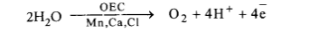# Mention the major events during

Question:

Mention the major events during photosynthesis. (CCE 2011, 2012)

Solution:

1. Photolysis: With the help of light energy, oxygen evolving Z-complex splits up water into its components - protons $\left(\mathrm{H}^{+}\right)$, electrons $\left(\mathrm{e}^{-}\right)$and oxygen.2. Absorption of Light Energy: Chlorophyll absorbs light energy.

3. Primary Reaction: Chlorophyll converts the absorbed light energy into chemical energy. It is called primary reaction of photosynthesis. It builds up ATP with the help of excited electrons.

4. Formation of Reducing Power: Coenzyme NADP $^{+}$is changed to reduced form of NADPH.

$\mathrm{NADP}^{+}+\mathrm{H}^{+}+2 \overline{\mathrm{e}} \rightarrow \mathrm{NADPH}$

5. Reduction of $\mathrm{CO}_{2}$ : Carbon dioxide is reduced enzymatically with the help of $\mathrm{NADPH}$ and ATP to form carbohydrates.

$6 \mathrm{CO}_{2}+12 \mathrm{NADPH}+18 \mathrm{ATP} \rightarrow \mathrm{C}_{6} \mathrm{H}_{12} \mathrm{O}_{6}+12 \mathrm{NADP}^{+}+18 \mathrm{ADP}+18 \mathrm{Pi}$